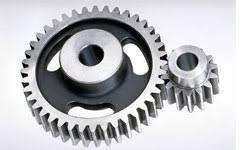# Types of Motion

## There are basically four types of motion in the world of mechanics.

These four types are:

1. Linear.
2. Reciprocating.
3. Rotary.
4. Oscillating.
Each one moves in a slightly different way, in addition each type of motion is achieved using a different mechanical means.  Lets go through each type of motion to help us understand each one in terms of mechanics and what this means to achieve each motion.

## Linear MotionLinear Motion is defined as a device that moves in a straight line, but can sometimes be called Translational motion. The Firgelli Linear Actuators are a classic example of Linear Motion.  The interesting part of Electro Mechanical Linear Motion Actuators however is that the straight line motion actually comes from rotary motion that we explain next.  In Linear Actuators they use AC or DC motors to create roary motion which gets converted via a Leadscrew into Linear motion.

## Rotary MotionIn Rotary Motion or Rotational motion, this refers to anything that goes in a circle and is further defined as a circular movement of an object around a center (or point) of rotation. Rotary Motion is perhaps the most common and important types of motion and also the most widely used in every part of our daily lives. From Engines, to fans to aircraft, you name it and just about everything has some form of rotary motion.

## How do you create Rotary Motion?

The most common way to create Rotary motion is using Motors, These could be AC or DC Electrical motors but could also be an internal combustion engine whos end result is to turn the wheels of a car. Interestingly however in an internal combustion engine the initial form of motion is actually linear. The engines cylinders use air and fuel to ignite which then explodes and forces the piston to move in a linear motion and then the piston is connected to a crank shaft to create rotary motion. So in this instance reciprocating motion is used to create rotary motion. The earth also rotates arounds its North and south poles axis once every 24 hours.

## Reciprocating MotionReciprocating Motion also called reciprocation, is a repetitive back-and-forth linear. It is found in a wide range of mechanisms, including internal combustions engines as mentioned above where it is used to create rotary motion. This type of motion can be driven from either end of the mechanism such as the linear motion end as used in an internal combustion engine that then converted into rotary motion. But it can also be driven from the rotary end where linear motion is the desired output.

## Oscillating Motion

Oscillatory motion is defined as the back and forth motion of an object from its mean position. When you describe mechanical oscillation, the term vibration is used which is found in a swinging pendulum type of motion.

## Type of Oscillating Motion

• Pendulum of a clock
• Playground swing
• Quantum harmonic oscillator
• A sprinkler system